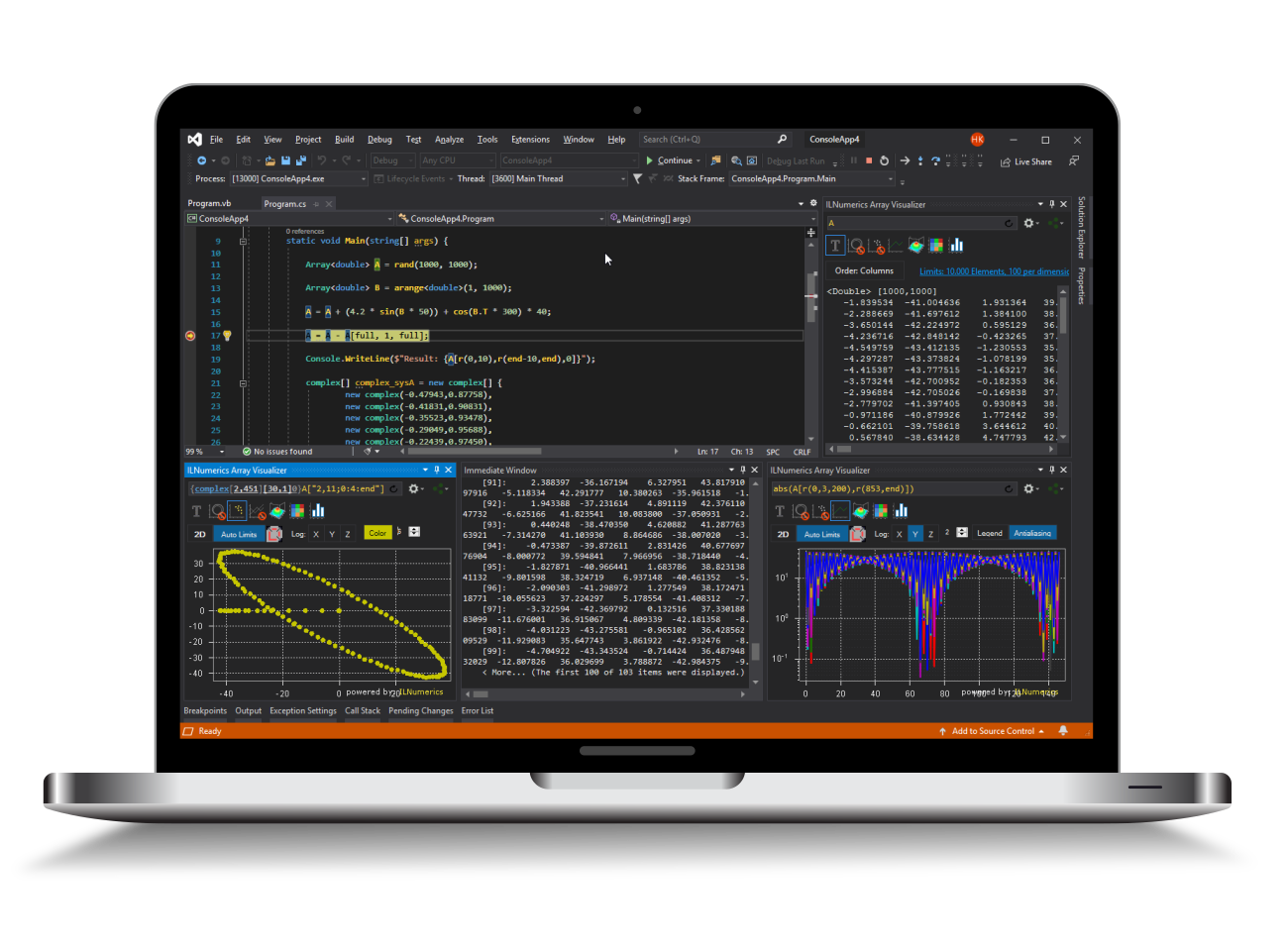Industrial Data Science
in C# and .NET:
Simple. Fast. Reliable.ILNumerics - Technical Computing

Modern High Performance Tools for Technical

Computing and Visualization in Industry and Science

tgt

# Newton-Raphson method in .NET (C# and Visual Basic)

The Newton-Raphson or Newton routine finds the unconstrained minimum of a scalar function. It's an iterative method that calculates the next point according to $$x_{n+1} = x_n \cdot \frac{f(x_n)}{f'(x_n)}$$ where $f$ is the function to be minimized, $f'$ its derivative and $x_i$ is the i-th point in the iteration. For more details see the Wikipedia article.